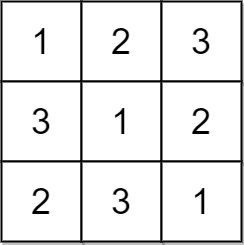Check if Every Row and Column Contains All Numbers Solution Leetcode

An n x n matrix is valid if every row and every column contains all the integers from 1 to n (inclusive).

Given an n x n integer matrix matrix, return true if the matrix is valid. Otherwise, return false.

Example 1: Check if Every Row and Column Contains All Numbers Solution LeetcodeInput: matrix = [[1,2,3],[3,1,2],[2,3,1]]
Output: true
Explanation: In this case, n = 3, and every row and column contains the numbers 1, 2, and 3.
Hence, we return true.

Example 2: Check if Every Row and Column Contains All Numbers Solution LeetcodeInput: matrix = [[1,1,1],[1,2,3],[1,2,3]]
Output: false
Explanation: In this case, n = 3, but the first row and the first column do not contain the numbers 2 or 3.
Hence, we return false.

Constraints:

• n == matrix.length == matrix[i].length
• 1 <= n <= 100
• 1 <= matrix[i][j] <= n